SYLLABUS  Previous: 2.5.1 Advection-diffusion equation  Up: 2.5 Implicit Crank-Nicholson  Next: 2.6 Computer quiz

2.5.2 Schrödinger equation

In quantum mechanics, the evolution of a particle is generally modelled with a complex wave function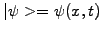. If the particle does't decay with time, the total probability of finding this particle somewhere in space of course remains exactly unity at all times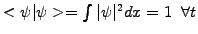. Solving the Schrödinger equation normalized to have Planck's constant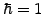, the particle mass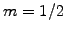and the static potential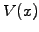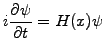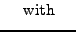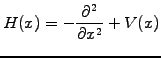(2.5.2#eq.13)

it is clearly an advantage to formulate a numerical scheme that conserves the probability by keeping the evolution operator unitary even as it is discretized. This can be achieved by writing a formal solution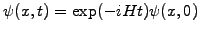in the so-called Cayley form with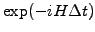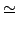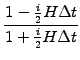(2.5.2#eq.14)

This approximation is unitary, accurate to second-order in time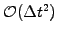. It suggests an evolution of the form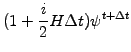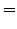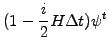(2.5.2#eq.15)

Replacing the second order derivative in the Hamiltonian operator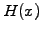with finite differences centered in space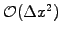, one obtains a scheme that is stable, unitary and is in fact the same Crank-Nicholson method applied here for the Schrödinger equation: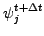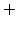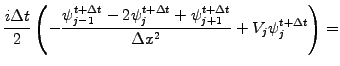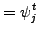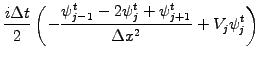(2.5.2#eq.16)

which is finally cast into the linear system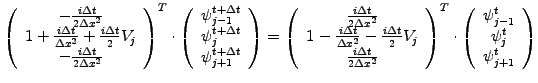(2.5.2#eq.17)

Exploiting the tri-diagonal structure of the matrix, the scheme has been implemented in JBONE using complex arithmetic
      BandMatrixC a = new BandMatrixC(3, h.length);  //Complex objects
BandMatrixC b = new BandMatrixC(3, h.length);
Complex[]   c = new Complex[h.length];
Complex     z = new Complex();
double[] V = physData.getPotential();          //Heavyside(x-L/2) times
double  scale  = 10.*velocity;                 //  an arb. scaling factor
double  dtodx2 = timeStep/(dx*dx);
Complex pih  = new Complex(0., 0.5*dtodx2);
Complex mih  = new Complex(0.,-0.5*dtodx2);
Complex pip1 = new Complex(1.,     dtodx2);
Complex mip1 = new Complex(1.,    -dtodx2);

for (int j=0; j<=n; j++) {
z = new Complex(0.,0.5*scale*timeStep*V[j]);
a.setL(j,mih);                               //Matrix elements
a.setR(j,mih);
b.setL(j,pih);                               //Right hand side
b.setD(j,mip1.sub(z));
b.setR(j,pih);
}
c=b.dot(h);                                    //Right hand side with
hp=a.solve3(c);                                //Solve linear problem

for (int j=0; j<=n; j++){                      //Plot norm & real part
fp[j]=hp[j].norm();
gp[j]=hp[j].re();
}

The code again relies on the complex BandMatrixC.solve3() method to solve the linear system efficiently with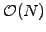complex operations using the standard LU-factorization that will be studied in 3.

The applet below illustrates the scheme with a calculation of the scattering of a wave packet on a square potential barrier.

The perfect conservation of the lowest order moment shows that the total probability is indeed conserved for all times.

 Numerical experiments: Crank-Nicholson for Schrödinger Modify the energy in the wavepacket ICWavelength and verify that the applet indeed reproduces the reflection / transmission coefficients that you expect. Increase the value of the TimeStep and explain what you observe. Extend the RunTime and observe how quasi-modes are formed, with reflections in the periodic domain that remind what happens to an electron in a crystal.

SYLLABUS  Previous: 2.5.1 Advection-diffusion equation  Up: 2.5 Implicit Crank-Nicholson  Next: 2.6 Computer quiz